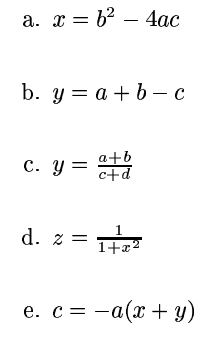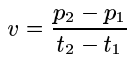# CMSC 104, Section 01, Fall 2016 Problem Solving and Computer Programming

## Project 4

Due Date: Tuesday, November 1st @ 5:30 PM

Point Value: This project is graded with a 75 point max score.

Objectives:

To become more familiar with:
• C program creation, compilation, and execution
• C programming syntax
• Pseudocode and problem solving
• Mathematical Formulas in C

For questions 1-3, place your answers in a text file named campusid_proj4.txt.

1. (15 points) Rewrite the following mathematical formulas in proper C programming format.2. (15 points) Given the following constants and variable declarations:
```#define PI 3.14
#define MAX_I 1000
...
double x, y = -1.0;
int a = 3, b = 4, i;
```
...indicate which of the following statements are valid and find the value stored by each valid statement. Additionally, indicate which are invalid and explain why. (Hint: if you are having trouble, you are encouraged to write code!)
```a.     i = a % (a / b);

b.     i = (MAX_I - 990) / a;

c.     x = b /a;

d.     x = a % (990 - MAX_I);

e.     i = a % (MAX_I - 990);
```

3. (20 points) Write a pseudocode algorithm that will prompt the user for input, then calculate and report the average velocity of a particle traveling along a line. The user should input the starting point p1, the ending point p2, the starting time t1, and the ending time t2. The formula for average velocity v of a particle traveling on a line between two points p1 and p2 in time t1 and t2 is4. (25 points) Create a C program, "campusid_velocity.c", that implements the pseudocode you wrote in #3. Input and output values should be floating point. As always, style is part of your grade, so be sure to follow proper formatting conventions as documented on the class webpage!

When you have finished, submit your campusid_proj4.txt and campusid_velocity.c files using the following commands:

curl -L -F file=@campusid_proj4.txt http://userpages.umbc.edu/~reicht1/classes/cmsc104/fall16/submit.php

curl -L -F file=@campusid_velocity.c http://userpages.umbc.edu/~reicht1/classes/cmsc104/fall16/submit.php

Additionally, email your campusid_proj4.txt and campusid_velocity.c files as an attachment to reicht1@umbc.edu using the subject line Project Submission - 4 - Lastname, Firstname, replacing Lastname, Firstname with your name.